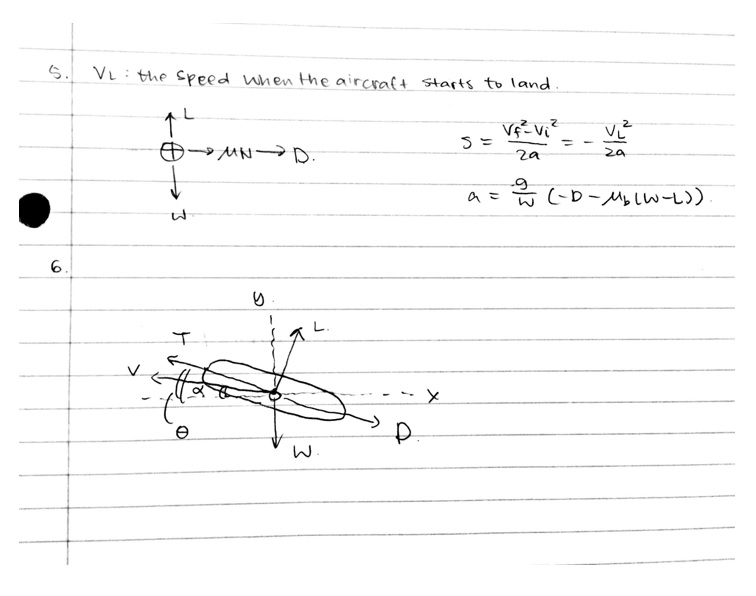Problem 1 (10 pts): Create an atmospheric spreadsheet (Excel or alike) that calculate theta, sigma, and delta. Use that spreadsheet to plot pressure, temperature, and density as a function of pressure altitude. Make sure to include ∆ISA (Off Standard Atmospheric Temperature) in Celsius as an input. What does that affect? Provide your plot and Excel file with your homework.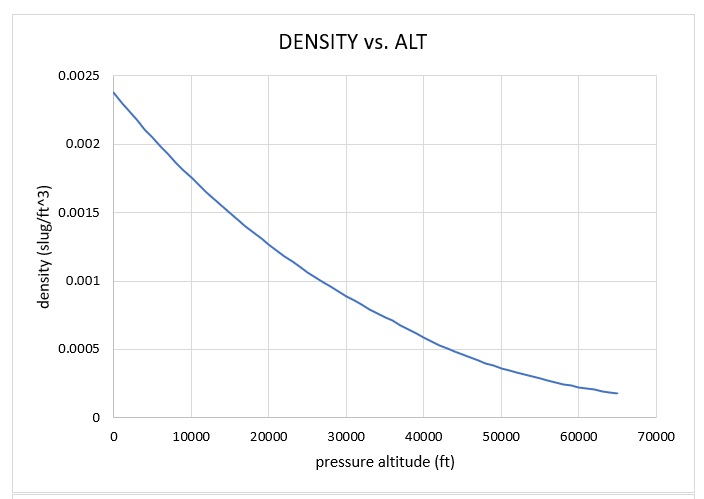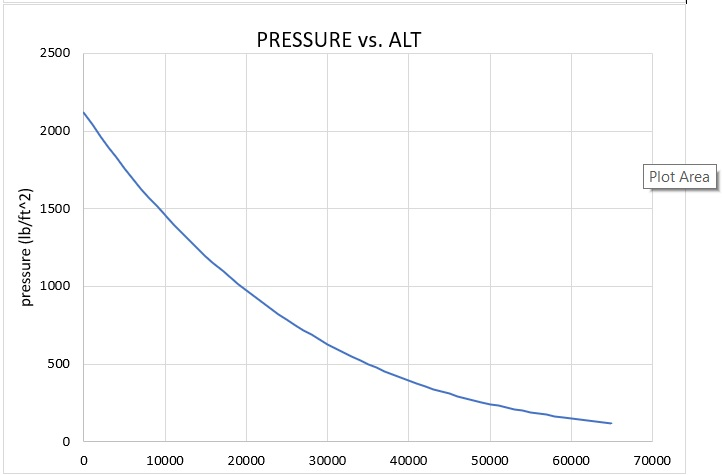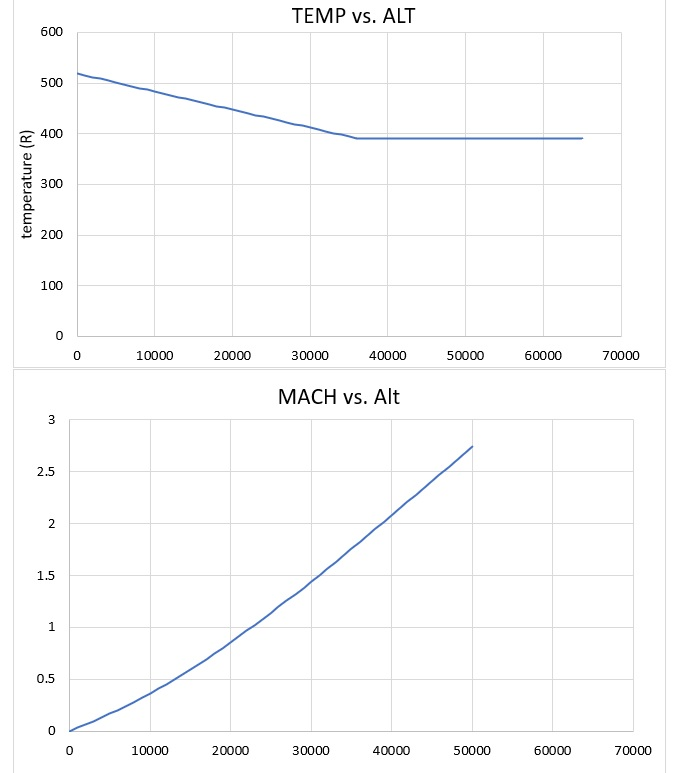∆ISA means the temperature relative to the International Standard Atmosphere, but we assume a standard day condition while ∆ISA is always zero, where the temperature is neither higher or lower than a standard temperature for the certain altitude.

Problem 2 (10 pts): In your atmospheric spreadsheet make sure to calculate:

• Mach Number based on Calibrated Airspeed
• Calibrated Airspeed based on Mach Number
• True airspeed On a pressure altitude vs Mach Number chart, plot lines of constant (choose your line spacing smartly)
• Calibrated airspeed from 0 to 700 KCAS
• Dynamic pressure from 0 to 1,500 psf

What do you notice about the lines? Do they follow a particular trend? How do the Differ? What about lines of Equivalent Airspeed? Provide your plot and Excel file with your homework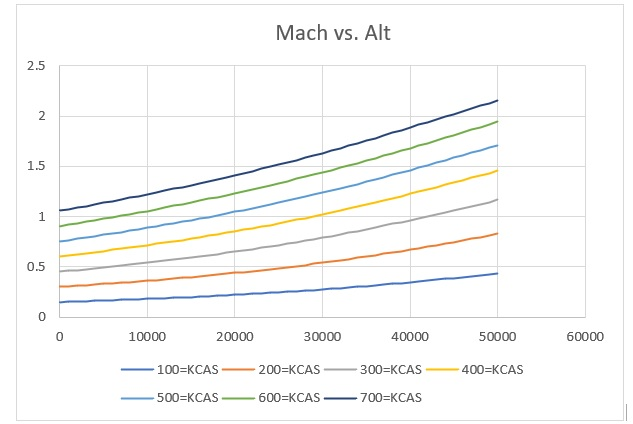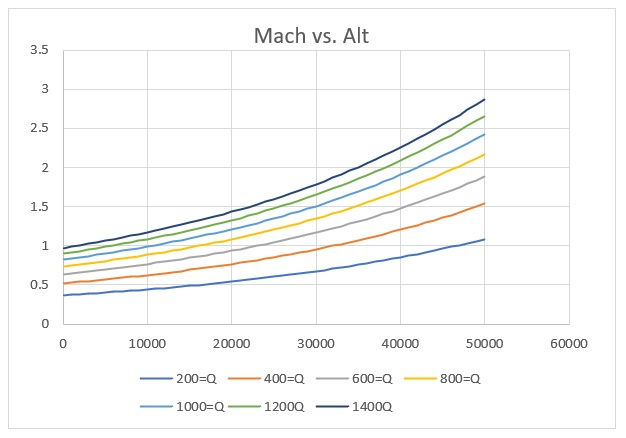All the lines have a positive slope with respect to increasing altitude. They follow the same increasing trend in response to increasing pressure altitude. Their differences lie in the slope number and trend shapes while the first graph has a more linear shape with lower slope. The lines of equivalent airspeed decrease with respect to altitude.

Problem 3 (20 pts): In a spreadsheet repeat the time step integration exercise from class using the provided data in Figure 1, Table 1, and Table 2. Assume the aircraft initiates cruise at 75,500 lb and Mach 0.85 at FL410 (41,000 ft) for 5,000 lbs of fuel and the climbs to FL430 (43,000 ft) for the remaining 5,000 lb, assuming the climb happens instantaneously. Compare to the Breguet range equation.

• How does climbing affect L/D?
• How does climbing affect SFC?
• What happens if the entire cruise happens at FL410?
• Why can’t the aircraft begin cruise at FL430?
• What conclusions/observations can you make? Provide your solutions and Excel file with your homework NOTE: This example does not reflect Reynolds number variations with altitude. This effect is typically ~1 count (1 count = 0.0001 CD) of cdo per 1000 ft. It can also be calculated using Reynolds Number.

Using Breguet Equation:

Using time integration: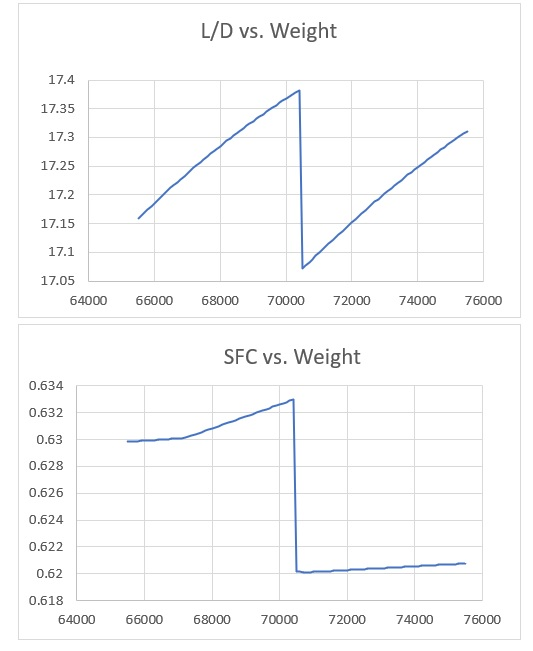According the graphs above, L/D increases while cruising, and during the instantaneous climb, L/D experiences a sudden drop, and then continues to increase afterwards, exceeding its initial value. SFC follows the similar pattern, where the value drops significantly during the climb, then catches up afterwards.

If the entire cruise happens at FL410, or 41,000 ft, the value for dynamic pressure and airspeed would stay the same throughout the entire trip since the altitude does not change and the ambient properties such as temperature and pressure remain the same.

The aircraft begin cruise at FL430, or 43,000 ft because the total weight is large initially or that the lift coefficient is high, along with the induced drag, which is also high. Thrust cannot overcome the high drag, leading to dangerous flight. High altitude should be reached within a weight limit.

Problem 4 (5 pts): Pick an aircraft and estimate the L/D and SFC using the Breguet Range equation. What assumptions did you have to make to determine SFC and/or L/D?

A380: (Trent 900):

Fuel Fraction ：44.2%

TSFC: 15.5 (g/s)/kN or 0.5 lb/lbf*hr

Max range: 8500 nm or 51646981.6 ft

Cruising speed: 490kt or 827.02683 ft / sec

K = 0.97

Solve for L/D to get 15.34

Assumptions should be made for cruising conditions, and others including maximum range and cruising speed, constant SFC and final weight with exhausted fuel.

Problem 5 (10 pts): Repeat the TOFL analysis from class for landing. What is landing field length proportional to? How is it different from TOFL.

Problem 6 (5 pts): Draw a free body diagram for an aircraft in climb. Label all forces and angles.

 https://en.wikipedia.org/wiki/Airbus_A380

 http://webserver.dmt.upm.es/~isidoro/bk3/c17/Aerospace%20engine%20data.pdf

 https://en.wikipedia.org/wiki/Airbus_A380

 https://en.wikipedia.org/wiki/Airbus_A380